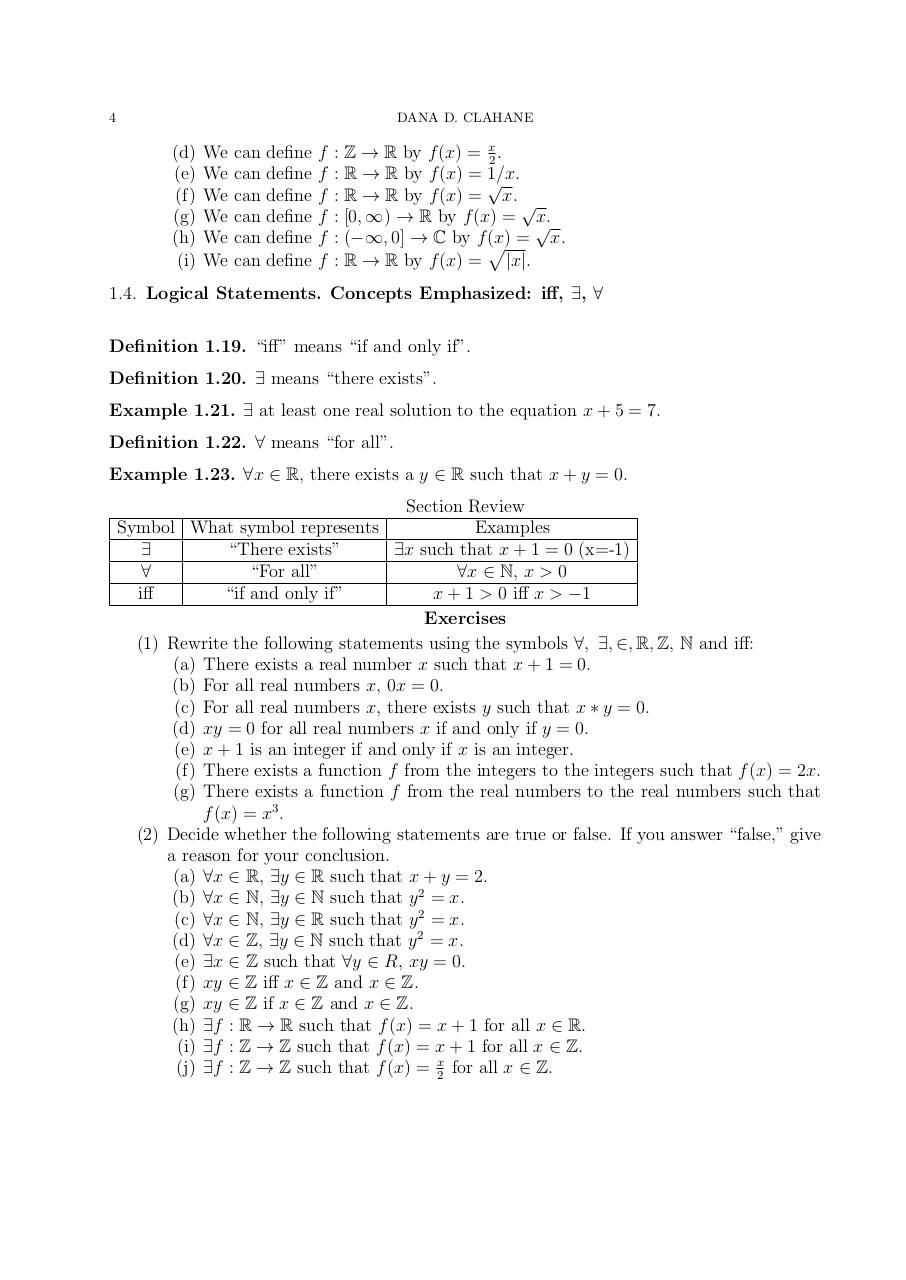# ODELAClahane061416.pdfPage 1 2 3 45670

#### Text preview

4

DANA D. CLAHANE

(d)
(e)
(f)
(g)
(h)
(i)

We
We
We
We
We
We

can
can
can
can
can
can

define
define
define
define
define
define

f
f
f
f
f
f

: Z → R by f (x) = x2 .
: R → R by f (x) = 1/x.

: R → R by f (x) = x. √
: [0, ∞) → R by f (x) = √
x.
: (−∞, 0] → C by f p
(x) = x.
: R → R by f (x) = |x|.

1.4. Logical Statements. Concepts Emphasized: iff, ∃, ∀
Definition 1.19. “iff” means “if and only if”.
Definition 1.20. ∃ means “there exists”.
Example 1.21. ∃ at least one real solution to the equation x + 5 = 7.
Definition 1.22. ∀ means “for all”.
Example 1.23. ∀x ∈ R, there exists a y ∈ R such that x + y = 0.
Section Review
Symbol What symbol represents
Examples

“There exists”
∃x such that x + 1 = 0 (x=-1)

“For all”
∀x ∈ N, x &gt; 0
iff
“if and only if”
x + 1 &gt; 0 iff x &gt; −1
Exercises
(1) Rewrite the following statements using the symbols ∀, ∃, ∈, R, Z, N and iff:
(a) There exists a real number x such that x + 1 = 0.
(b) For all real numbers x, 0x = 0.
(c) For all real numbers x, there exists y such that x ∗ y = 0.
(d) xy = 0 for all real numbers x if and only if y = 0.
(e) x + 1 is an integer if and only if x is an integer.
(f) There exists a function f from the integers to the integers such that f (x) = 2x.
(g) There exists a function f from the real numbers to the real numbers such that
f (x) = x3 .
(2) Decide whether the following statements are true or false. If you answer “false,” give